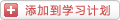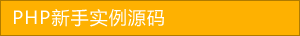﻿ PHP行为设计模式之策略模式 - php高级应用 - PHP粉丝网

# PHP行为设计模式之策略模式

##### 发布:smiling　来源: PHP粉丝网 　添加日期：2023-07-06 16:43:58　浏览: 评论：0

PHP策略模式（Strategy Pattern）

1. <?php
2. interface MathStrategy
3.     public function calculate(int \$a, int \$b): int;
5.     public function calculate(int \$a, int \$b): int
6.     {
7.         return \$a + \$b
8.     }
9. class Multiplication implements MathStrategy
10.     public function calculate(int \$a, int \$b): int
11.     {
12.         return \$a * \$b
13.     }
14. class MathContext
15.     private \$strategy
16.     public function __construct(MathStrategy \$strategy
17.     {
18.         \$this->strategy = \$strategy
19.     }
20.     public function setStrategy(MathStrategy \$strategy): void
21.     {
22.         \$this->strategy = \$strategy
23.     }
24.     public function calculate(int \$a, int \$b): int
25.     {
26.         return \$this->strategy->calculate(\$a\$b);
27.     }
28. \$context = new MathContext(new Addition());
29. echo \$context->calculate(2, 3) . "\n"// output: 5
30. \$context->setStrategy(new Multiplication());
31. echo \$context->calculate(2, 3) . "\n"// output: 6

5

6

### 分享到： document.write('<a href="http://v.t.sina.com.cn/share/share.php?url='+encodeURIComponent(location.href)+'&appkey=3172366919&title='+encodeURIComponent('PHP行为设计模式之策略模式')+'" title="分享到新浪微博" class="t1" target="_blank">&nbsp;</a>'); document.write('<a href="http://www.douban.com/recommend/?url='+encodeURIComponent(location.href)+'&title='+encodeURIComponent('PHP行为设计模式之策略模式')+'" title="分享到豆瓣" class="t2" target="_blank">&nbsp;</a>'); document.write('<a href="http://share.renren.com/share/buttonshare.do?link='+encodeURIComponent(location.href)+'&title='+encodeURIComponent('PHP行为设计模式之策略模式')+'" title="分享到人人" class="t3" target="_blank">&nbsp;</a>'); document.write('<a href="http://www.kaixin001.com/repaste/share.php?rtitle='+encodeURIComponent('PHP行为设计模式之策略模式')+'&rurl='+encodeURIComponent(location.href)+'&rcontent=" title="分享到开心网" class="t4" target="_blank">&nbsp;</a>'); document.write('<a href="http://sns.qzone.qq.com/cgi-bin/qzshare/cgi_qzshare_onekey?url='+encodeURIComponent(location.href)+'" title="分享到QQ空间" class="t5" target="_blank">&nbsp;</a>');